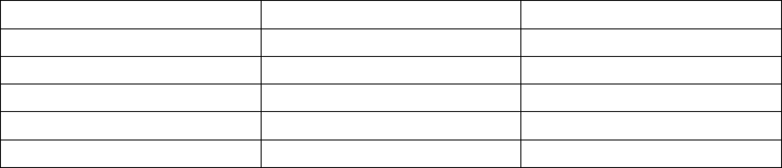# ECON 208 Chapter Notes - Chapter 3: Ceteris Paribus, Takers, Inferior Good

33 views3 pages
Page:
of 3Chapter 3 Demand, Supply, and Price
Sept.18.12
Quantity demanded:
- Total amount that consumers desire to purchase in some time period
- Quantity bought/exchanged refers to actual purchases
- Quantity demanded is a flow variable
- Influenced by:
- products’ own price
- consumers’ income
- prices of other products
- tastes
- population
- expectations about the future
Demand schedules and demand curves:
- Ask consumers how much they would like to buy at each given price before
the amount is produced
- Not the actual amount of what will be exchanged in the market
Price per ton
Quantity demanded
U
\$20
110
V
\$40
85
W
\$60
65
X
\$80
50
Y
\$100
40
Distinction between stocks and flows:
- Flow variable: it is so much per unit of time
- Stock variable: has a meaning at a point in time
- i.e. income = flow, consumer expenditure = flow, amount of money in a bank
account = stock
Quantity demanded and price:
- Basic hypothesis - ceteris paribus: price of a product and the quantity
demanded are negatively related
- There are usually several products that can satisfy any given want or desire
- Reducing the price of a product means that the specific desire can now be
satisfied more cheaply by buying more of that product
- Effect on demand due to an increase in household income:
1. Normal good: quantity demanded increases when income rises
2. Inferior good: quantity demanded falls when income rises
- Distribution of income: changes for particular goods
- Prices of other products: substitutes vs. complements
- Change in demand = change in quantity demanded at every price a shift of
the entire curve
- Change in quantity demanded = refers to a movement from one point on a
demand curve to another point either on the same demand curve or on a
new one
Supply:
- When price increases so does supply
- Changes in price imply moves along the supply curve
- Changes in variables other than the price shift the whole curve right or left
- prices of inputs
- technology
- government taxes/subsidies
- prices of other products (substitutes and complements)
- number of suppliers entering and exiting the market
Supply schedules:
Price per ton
Quantity supplied
U
\$20
20
V
\$40
45
W
\$60
65
X
\$80
80
Y
\$100
95
Changes in the prices of other products: substitutes vs. complements:
Demand side:
1. price of substitute goes up demand increases
2. price of complement goes up demand decreases
Supply side:
1. price of substitute goes up supply decreases
2. price of complement increases supply increases
Determination of price:
- Concept of a market
- any situation in which buyers and sellers negotiate the transaction of
goods/services
- may differ in the degree of competition among the buyers and sellers
- in perfectly competitive markets buyers and sellers are price takers
Comparative statics:
- Determine how the endogenous variables will change following a change in
some exogenous variables (why curves shift)
- Procedure:
- equilibrium position
- introduce the change to be studied (shift in supply/demand curve)
- determine new equilibrium position
- compare with original
- difference between the two positions of equilibrium is a result of the
change introduced because every other variable was held constant
- Effects of four possible curve shifts:
1. Increase in demand (right shift): increase in equilibrium price and the
equilibrium quantity exchanged
2. Decrease in demand (left shift): decrease in equilibrium price and the
equilibrium quantity exchanged
3. Increase in supply (right shift): decrease in equilibrium price and increase
in the equilibrium quantity exchanged
4. Decrease in supply (left shift): increase in equilibrium price and a decrease
in the equilibrium quantity exchanged
Relative prices and inflation:
- Relative price: ratio of the price of one commodity to the price of another
commodity
- Absolute price: the amount of money that must be spent to acquire one unit
of a commodity
- i.e. if the price of carrots rises while the price of other vegetables remains
constant, consumers will reduce their quantity demanded of carrots, then
the relative price of carrots has increased
- i.e. if the price of carrots rises while the price of other vegetables rises too
then the relative price of carrots is constant
- i.e. if the price of carrots falls while the price of other vegetables rises then
the carrots have become relatively cheap